# DOMV No 4 PHYSICAL DYNAMIC MODEL TYPES (1).pdf

30 May 2023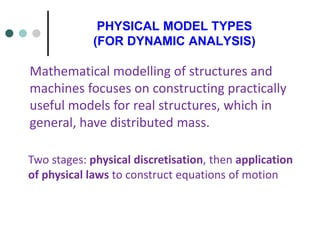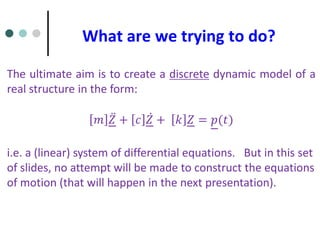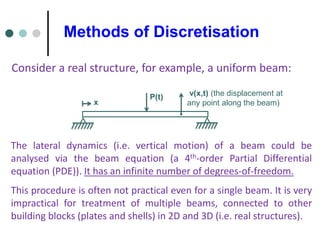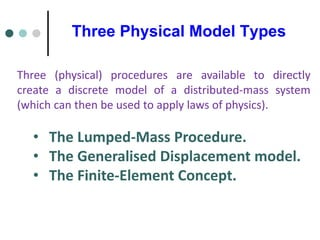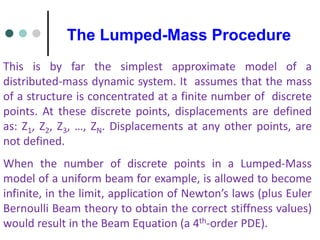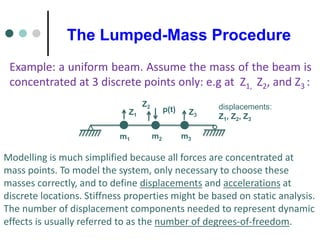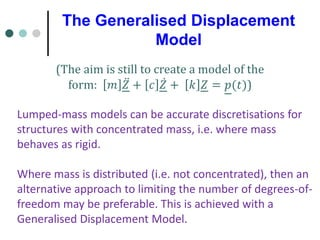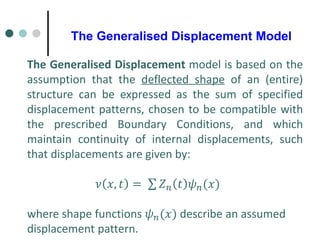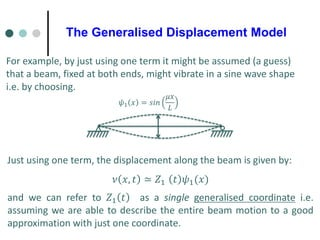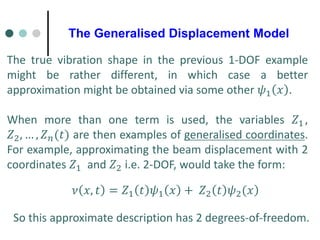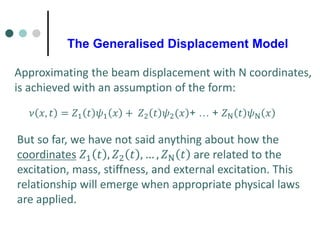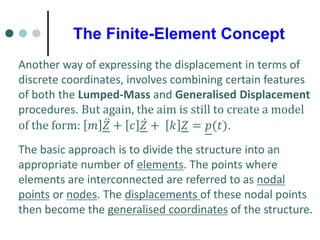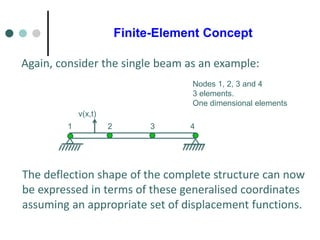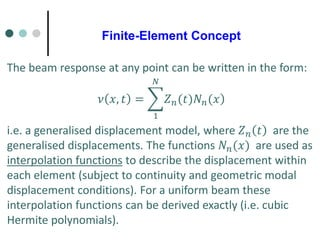1 sur 14

### DOMV No 4 PHYSICAL DYNAMIC MODEL TYPES (1).pdf

• 1. PHYSICAL MODEL TYPES (FOR DYNAMIC ANALYSIS) Mathematical modelling of structures and machines focuses on constructing practically useful models for real structures, which in general, have distributed mass. Two stages: physical discretisation, then application of physical laws to construct equations of motion
• 2. What are we trying to do? The ultimate aim is to create a discrete dynamic model of a real structure in the form: 𝑚 ሷ 𝑍 + 𝑐 ሶ 𝑍 + 𝑘 𝑍 = 𝑝(𝑡) i.e. a (linear) system of differential equations. But in this set of slides, no attempt will be made to construct the equations of motion (that will happen in the next presentation).
• 3. Methods of Discretisation Consider a real structure, for example, a uniform beam: ν(x,t) (the displacement at any point along the beam) x P(t) The lateral dynamics (i.e. vertical motion) of a beam could be analysed via the beam equation (a 4th-order Partial Differential equation (PDE)). It has an infinite number of degrees-of-freedom. This procedure is often not practical even for a single beam. It is very impractical for treatment of multiple beams, connected to other building blocks (plates and shells) in 2D and 3D (i.e. real structures).
• 4. Three Physical Model Types Three (physical) procedures are available to directly create a discrete model of a distributed-mass system (which can then be used to apply laws of physics). • The Lumped-Mass Procedure. • The Generalised Displacement model. • The Finite-Element Concept.
• 5. The Lumped-Mass Procedure This is by far the simplest approximate model of a distributed-mass dynamic system. It assumes that the mass of a structure is concentrated at a finite number of discrete points. At these discrete points, displacements are defined as: Z1, Z2, Z3, …, ZN. Displacements at any other points, are not defined. When the number of discrete points in a Lumped-Mass model of a uniform beam for example, is allowed to become infinite, in the limit, application of Newton’s laws (plus Euler Bernoulli Beam theory to obtain the correct stiffness values) would result in the Beam Equation (a 4th-order PDE).
• 6. The Lumped-Mass Procedure p(t) m1 m2 m3 Z1 Z2 Z3 displacements: Z1, Z2, Z3 Example: a uniform beam. Assume the mass of the beam is concentrated at 3 discrete points only: e.g at Z1, Z2, and Z3 : Modelling is much simplified because all forces are concentrated at mass points. To model the system, only necessary to choose these masses correctly, and to define displacements and accelerations at discrete locations. Stiffness properties might be based on static analysis. The number of displacement components needed to represent dynamic effects is usually referred to as the number of degrees-of-freedom.
• 7. The Generalised Displacement Model (The aim is still to create a model of the form: 𝑚 ሷ 𝑍 + 𝑐 ሶ 𝑍 + 𝑘 𝑍 = 𝑝(𝑡)) Lumped-mass models can be accurate discretisations for structures with concentrated mass, i.e. where mass behaves as rigid. Where mass is distributed (i.e. not concentrated), then an alternative approach to limiting the number of degrees-of- freedom may be preferable. This is achieved with a Generalised Displacement Model.
• 8. The Generalised Displacement Model The Generalised Displacement model is based on the assumption that the deflected shape of an (entire) structure can be expressed as the sum of specified displacement patterns, chosen to be compatible with the prescribed Boundary Conditions, and which maintain continuity of internal displacements, such that displacements are given by: 𝜈 𝑥, 𝑡 = σ 𝑍𝑛 𝑡 𝜓𝑛(𝑥) where shape functions 𝜓𝑛(𝑥) describe an assumed displacement pattern.
• 9. The Generalised Displacement Model For example, by just using one term it might be assumed (a guess) that a beam, fixed at both ends, might vibrate in a sine wave shape i.e. by choosing. 𝜓1 𝑥 = 𝑠𝑖𝑛 𝜇𝑥 𝐿 Just using one term, the displacement along the beam is given by: 𝜈 𝑥, 𝑡 ≃ 𝑍1 𝑡 𝜓1(𝑥) and we can refer to 𝑍1 𝑡 as a single generalised coordinate i.e. assuming we are able to describe the entire beam motion to a good approximation with just one coordinate.
• 10. The Generalised Displacement Model The true vibration shape in the previous 1-DOF example might be rather different, in which case a better approximation might be obtained via some other 𝜓1 𝑥 . When more than one term is used, the variables 𝑍1 , 𝑍2, … , 𝑍𝑛(𝑡) are then examples of generalised coordinates. For example, approximating the beam displacement with 2 coordinates 𝑍1 and 𝑍2 i.e. 2-DOF, would take the form: ) 𝜈 𝑥, 𝑡 = 𝑍1 𝑡 𝜓1 𝑥 + 𝑍2 𝑡 𝜓2(𝑥 So this approximate description has 2 degrees-of-freedom.
• 11. The Generalised Displacement Model But so far, we have not said anything about how the coordinates 𝑍1 𝑡 , 𝑍2 𝑡 , … , 𝑍N 𝑡 are related to the excitation, mass, stiffness, and external excitation. This relationship will emerge when appropriate physical laws are applied. ) 𝜈 𝑥, 𝑡 = 𝑍1 𝑡 𝜓1 𝑥 + 𝑍2 𝑡 𝜓2(𝑥 + … + 𝑍N 𝑡 𝜓N 𝑥 Approximating the beam displacement with N coordinates, is achieved with an assumption of the form:
• 12. The Finite-Element Concept Another way of expressing the displacement in terms of discrete coordinates, involves combining certain features of both the Lumped-Mass and Generalised Displacement procedures. But again, the aim is still to create a model of the form: 𝑚 ሷ 𝑍 + 𝑐 ሶ 𝑍 + 𝑘 𝑍 = 𝑝(𝑡). The basic approach is to divide the structure into an appropriate number of elements. The points where elements are interconnected are referred to as nodal points or nodes. The displacements of these nodal points then become the generalised coordinates of the structure.
• 13. Finite-Element Concept Nodes 1, 2, 3 and 4 3 elements. One dimensional elements 1 ν(x,t) 2 3 4 Again, consider the single beam as an example: The deflection shape of the complete structure can now be expressed in terms of these generalised coordinates assuming an appropriate set of displacement functions.
• 14. Finite-Element Concept The beam response at any point can be written in the form: i.e. a generalised displacement model, where 𝑍𝑛 𝑡 are the generalised displacements. The functions 𝑁𝑛(𝑥) are used as interpolation functions to describe the displacement within each element (subject to continuity and geometric modal displacement conditions). For a uniform beam these interpolation functions can be derived exactly (i.e. cubic Hermite polynomials). 𝜈 𝑥, 𝑡 = ෍ 1 𝑁 ) 𝑍𝑛(𝑡)𝑁𝑛(𝑥